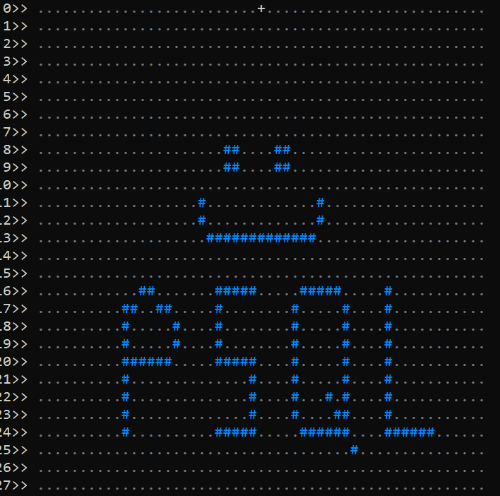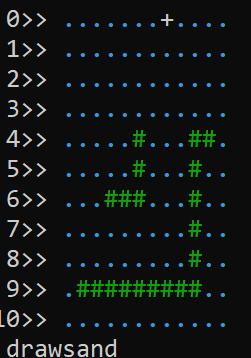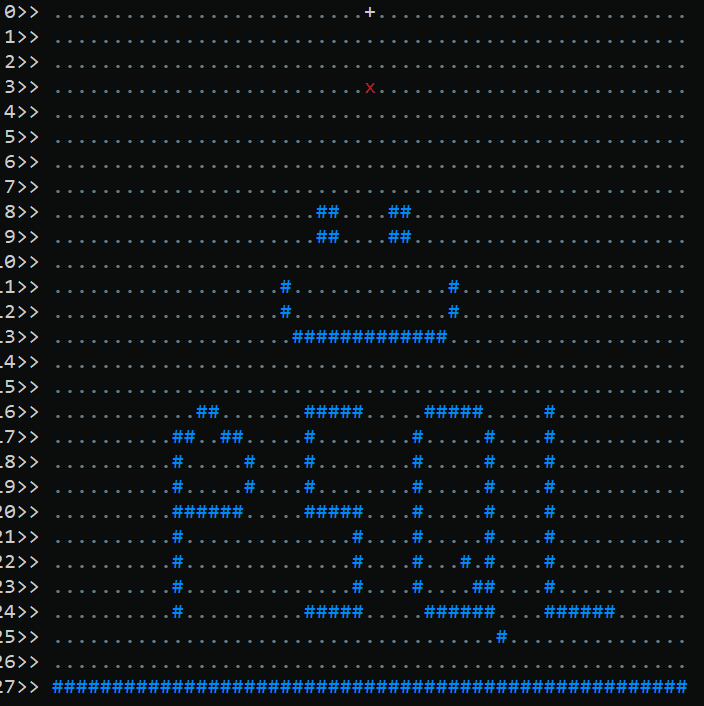Tutorial Instructions

# Advent of Code - Day 14

For this challenge, there are grains of sand falling from the top of a chamber. These grains fall onto various rock formations, and pile up on them, until they eventually spill onto the floor. We are tasked with figuring out how many grains fall before they spill, given dimensions about the rock formations. We are going to use a handful of tech for this, including:

file_fdw to turn our input file into a table

A sequence to enable simple counting of items

The string_to_table() and split_part() functions to help us transform the text input to usable parts

The lag() function to let us work with two rows at once

The generate_series() function to allow population of a table in a certain range of values

Our input file is a string of numbers denoting the edges of some rocks, and each line looks like this:

``484,24 -> 484,20 -> 489,20 -> 484,20 -> 484,17 -> 485,17``

The text file I received for the challenge is quite long, so I created my own. If you want to follow along, you can create it like so:

``````DROP FOREIGN TABLE if exists aoc_day14;
CREATE UNLOGGED TABLE aoc_day14(line text);
INSERT INTO aoc_day14 VALUES ('484,24 -> 484,20 -> 489,20 -> 484,20 -> 484,17 -> 485,17');
INSERT INTO aoc_day14 VALUES ('486,16 -> 487,16');
INSERT INTO aoc_day14 VALUES ('488,17 -> 489,17');
INSERT INTO aoc_day14 VALUES ('490,18 -> 490,19');
INSERT INTO aoc_day14 VALUES ('499,16 -> 495,16 -> 495,20 -> 499,20 -> 499,24 -> 495,24');
INSERT INTO aoc_day14 VALUES ('505,16 -> 509,16');
INSERT INTO aoc_day14 VALUES ('510,17 -> 510,24 -> 509,23');
INSERT INTO aoc_day14 VALUES ('509,24 -> 505,24');
INSERT INTO aoc_day14 VALUES ('504,23 -> 504,17');
INSERT INTO aoc_day14 VALUES ('511,25 -> 511,25');
INSERT INTO aoc_day14 VALUES ('508,22 -> 508,22');
INSERT INTO aoc_day14 VALUES ('515,16 -> 515,24 -> 520,24');
INSERT INTO aoc_day14 VALUES ('493,11 -> 493,12');
INSERT INTO aoc_day14 VALUES ('494,13 -> 506,13');
INSERT INTO aoc_day14 VALUES ('507,12 -> 507,11');
INSERT INTO aoc_day14 VALUES ('496,8 -> 497,9');
INSERT INTO aoc_day14 VALUES ('502,8 -> 503,9');``````

We want to get everything into a grid, so let's make a simple table to hold each point and what it contains:

``````DROP TABLE if exists sandgrid;
CREATE UNLOGGED TABLE sandgrid (
row int,
col int,
item text
);
CREATE UNIQUE INDEX sandslot ON sandgrid(row,col);
``````

First we break the input lines into separates rocks (one per line), and divide into the edges like so:

``````WITH x AS (SELECT nextval('aoc') AS rock, line FROM aoc_day14)
SELECT rock, string_to_table(line, ' -> ') AS points FROM x LIMIT 10;``````

`````` rock | points
------+--------
1 | 484,24
1 | 484,20
1 | 489,20
1 | 484,20
1 | 484,17
1 | 485,17
2 | 486,16
2 | 487,16
3 | 488,17
3 | 489,17
(10 rows)``````

The next step is to turn these numbers, which represent corners/vertices, into a full grid, where all the points between the vertices are represented. This is a little tricky, as there are a lot of numbers between the corners of the rock that need to be drawn. So we need to find every two vertices, then find a way to generate all the numbers between them. That is definitely a job for the `generate_series()` function. Because we also need to look at the current and the "previous" corner, we will use the window function `lag()` to be able to see both at once. For each pair of points, we will figure out the start and stop point in both grid directions (row and col), then use those numbers to fill in all the grid items between them. As we need those min and max entries to be generated and referenced for every point, we make sure they are called with `lateral`. Finally, we stick them all into our new table, using `distinct` to remove any duplicate entries:

``````WITH x AS (SELECT nextval('aoc') AS rock, line FROM aoc_day14)
,y AS (SELECT rock, string_to_table(line, ' -> ') AS ypoint FROM x)
,z AS (SELECT *, split_part(ypoint, ',', 1)::int AS xx, split_part(ypoint, ',', 2)::int AS yy FROM y)
,c AS (SELECT *, lag(xx) over () AS lagxx, lag(yy) over() AS lagyy, lag(rock) over() AS lagrock FROM z)
,xrows AS (
SELECT col, row FROM c
,LATERAL (SELECT row FROM generate_series(least(yy,lagyy),greatest(yy,lagyy)) row) i
,LATERAL (SELECT col FROM generate_series(least(xx,lagxx),greatest(xx,lagxx)) col) h
WHERE rock = lagrock
)
INSERT INTO sandgrid (row,col,item) SELECT distinct row,col,'#' FROM xrows;``````

(Yes, that was a lot to take in!) The puzzle also suggests using a period to represent places without a rock, i.e. "air", so we can use an upsert ("insert or update") statement to populate the rest of our grid:

``````WITH minmax AS ( /* get the boundaries of our current grid */
SELECT
min(row) AS minr, max(row) AS maxr,
min(col) AS minc, max(col) AS maxc
FROM sandgrid)
INSERT INTO sandgrid (row,col,item)
SELECT x,y,'.' FROM minmax, generate_series(0,maxr+1) AS x, generate_series(minc-1,maxc+1) AS y
ON CONFLICT DO NOTHING; /* ignore if this grid spot already has something */

/* Specify the source of the grains of sand: */
UPDATE sandgrid SET item = '+' WHERE row=0 AND col=500;``````

As a final sanity check, let's make a quick visualization function so our human brains can better see what the data in the table looks like:

``````CREATE or replace FUNCTION drawsand(xrow int, xcol int, herechar char default 'x')
returns void language plpgsql as \$\$
DECLARE
myrec record; output text = ''; myitem text;
BEGIN
/* Slow things down slightly. Comment out for max speed */
PERFORM pg_sleep(0.1);
/* For now, just hardcode the rows to view */
FOR x IN 0..27 LOOP
/* Add a newline for a clean start, then show the row number */
output = output || format('%s%2s>> ', chr(10), x);
/* Show a sensible number of columns */
FOR y IN 474..526 LOOP
/* See what is at this point */
SELECT INTO myitem item FROM sandgrid WHERE row=x AND col=y;

/* If this does not exist yet, just treat as 'air' */
IF myitem is null THEN myitem = '.'; END IF;

/* Output the current character, with a specific color */
IF x=xrow AND y=xcol THEN /* falling sand */
output = output || format(E'\033[31m%s\033[0m', herechar);
ELSIF '#' = myitem OR '-' = myitem THEN /* rock */
output = output || format(E'\033[38;5;33m%s\033[0m', myitem);
ELSIF '.' = myitem THEN /* air */
output = output || format(E'\033[38;5;8m%s\033[0m', myitem);
ELSIF 'o' = myitem THEN /* stuck sand */
output = output || format(E'\033[38;5;221m%s\033[0m', myitem);
ELSE /* source of sand */
output = output || format('%s', myitem);
END IF;
END LOOP;
END LOOP;
/* Clear the screen, then write out the string we have built */
RAISE NOTICE '% %', E'\033c', output;
return;
END;
\$\$;``````

Now we can see how it looks before we start dropping any grains of sand:

``SELECT drawsand(0,0);``Now we can write a function to implement the steps of the challenge. We release a grain of sand that falls downward due to gravity, then goes left if possible, then right is possible, until it cannot move further. At that point, the next grain of sand falls. Our job is to figure out how many grains of sand fall before the they start falling off the "edge" of the grid.

``````CREATE or replace FUNCTION sandflow(drawit bool default false)
returns int language plpgsql as \$\$
DECLARE
mincol int; maxcol int; maxrow int;
xrow int; xcol int; moved bool;
mychar char = 'x'; grains int = 0;
BEGIN
SELECT INTO mincol,maxcol,maxrow
min(col),max(col),max(row) FROM sandgrid WHERE item = '#';
<<mainloop>> FOR x IN 1..5432 LOOP
xrow = 0; xcol = 500; moved = false; grains = grains + 1;
<<grain>> LOOP
/* Can it go down? Future optimization: move down multiple steps */
PERFORM 1 FROM sandgrid WHERE col=xcol AND row=xrow+1 AND item = '.';
IF found THEN xrow = xrow + 1; moved = true;
ELSE
/* Cannot go down, so try diagonal left */
PERFORM 1 FROM sandgrid WHERE col=xcol-1 AND row=xrow+1 AND item = '.';
IF found THEN xrow = xrow + 1; xcol = xcol - 1; moved = true;
ELSE
/* Cannot go diagonal left, so try diagonal right */
PERFORM 1 FROM sandgrid WHERE col=xcol+1 AND row=xrow+1 AND item = '.';
IF found THEN xrow = xrow + 1; xcol = xcol + 1; moved = true;
ELSE EXIT; /* move to the next grain */
END IF;
END IF;
END IF; /* end check down ok, then left/right */
IF drawit THEN PERFORM drawsand(xrow,xcol,mychar); END IF;
CONTINUE grain; /* let this grain try and move again */
END LOOP; /* end of <<grain>> */
IF moved THEN
IF xrow > maxrow OR xcol=mincol OR xcol>maxcol THEN
mychar = '~';
IF drawit THEN continue; END IF;
EXIT;
END IF;
/* The sand grain has settled in place */
UPDATE sandgrid SET item = 'o' WHERE row=xrow and col=xcol;
ELSE
RAISE NOTICE '(%,% at %) Cannot move at all', xrow, xcol, x;
EXIT;
END IF;
END LOOP;
UPDATE sandgrid SET item='.' WHERE item='o';
return grains;
END;
\$\$;``````

For the function, we have two versions depending on the only argument. If you give it a `true` it will draw the state of the sandgrid table after every grain has moved. The test input file given by AOC will produce something like this:If we call it with just a `false` argument, we will get the correct answer:

``SELECT sandflow(false);``
``````sandflow
----------
51``````

For the second part of the challenge, the rules change a little bit. Now there is a "floor" of infinite dimensions, and we need to determine how many grains fall until things get clogged up and no more grains can fall. For this, we can use the exact same `sandflow` function, we just need to modify the map and add a floor. So we'll expand our whole grid a little bit, then add the floor at the bottom:

``````WITH minmax AS (SELECT min(row) AS minr, max(row) AS maxr,
min(col) AS minc, max(col) AS maxc FROM sandgrid)
INSERT INTO sandgrid (row,col,item)
SELECT x,y,'.' FROM minmax,
generate_series(minr,maxr+1) as x,
generate_series(minc-25,minc+46) as y
ON CONFLICT DO NOTHING;

UPDATE sandgrid SET item = '#' WHERE row = (SELECT max(row) FROM sandgrid);``````

Upon running, the total grains has jumped quite a bit:

``SELECT sandflow(false);``
``````NOTICE:  (0,500 at 543) Cannot move at all
sandflow
----------
543``````

That's all for today's challenge! Stay tuned for more. I will leave you with the output of running the same function, but with a `true` argument, which adds some nice animation: